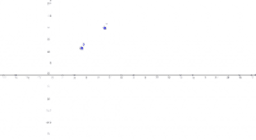•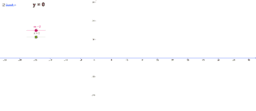### Exploring Linear Equations

Activity

•### Exploring Linear Equations

Activity

•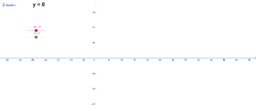### Exploring Linear Equations

Activity

•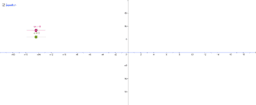### Linear Equations y= mx + c

Activity

•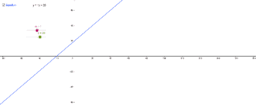### Linear Equations

Activity

•Activity

•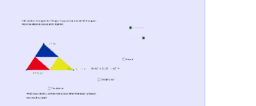•